# Six things I always Google when using ggplot2

My frequently-used reference for styling {ggplot2} charts.

reference
Published

January 27, 2020

I often use {ggplot2} to create graphs but there are certain things I always have to Google. I figured I’d create a post for quick reference for myself but I’d love to hear what you always have to look up!

To showcase what’s happening, I am going to use a TidyTuesday dataset: Spotify songs! Let’s start by creating a simple graph.

``````library(tidyverse)

spotify_songs <-

spotify_songs %>%
ggplot(aes(x = playlist_genre)) +
geom_histogram(stat = "count")``````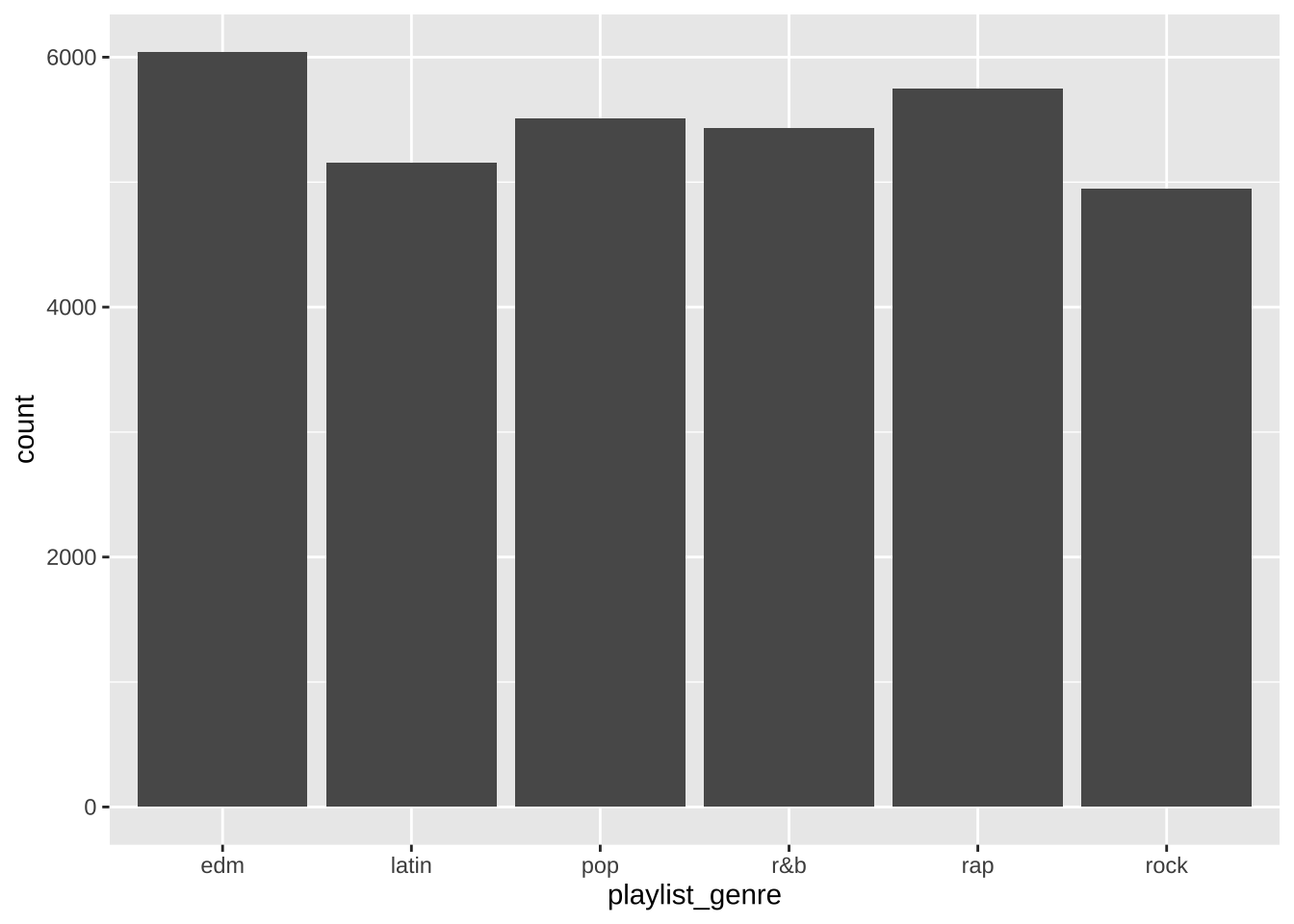## Remove the legend

`theme(legend.position = "none")`

Ahh… this one always gets me. Sometimes when your color is mostly just for aesthetics, it doesn’t make sense to also have a color legend. This removes the legend and makes the graph look cleaner.

``````spotify_songs %>%
ggplot(aes(x = playlist_genre, fill = playlist_genre)) +
geom_histogram(stat = "count") +
theme(legend.position = "none")``````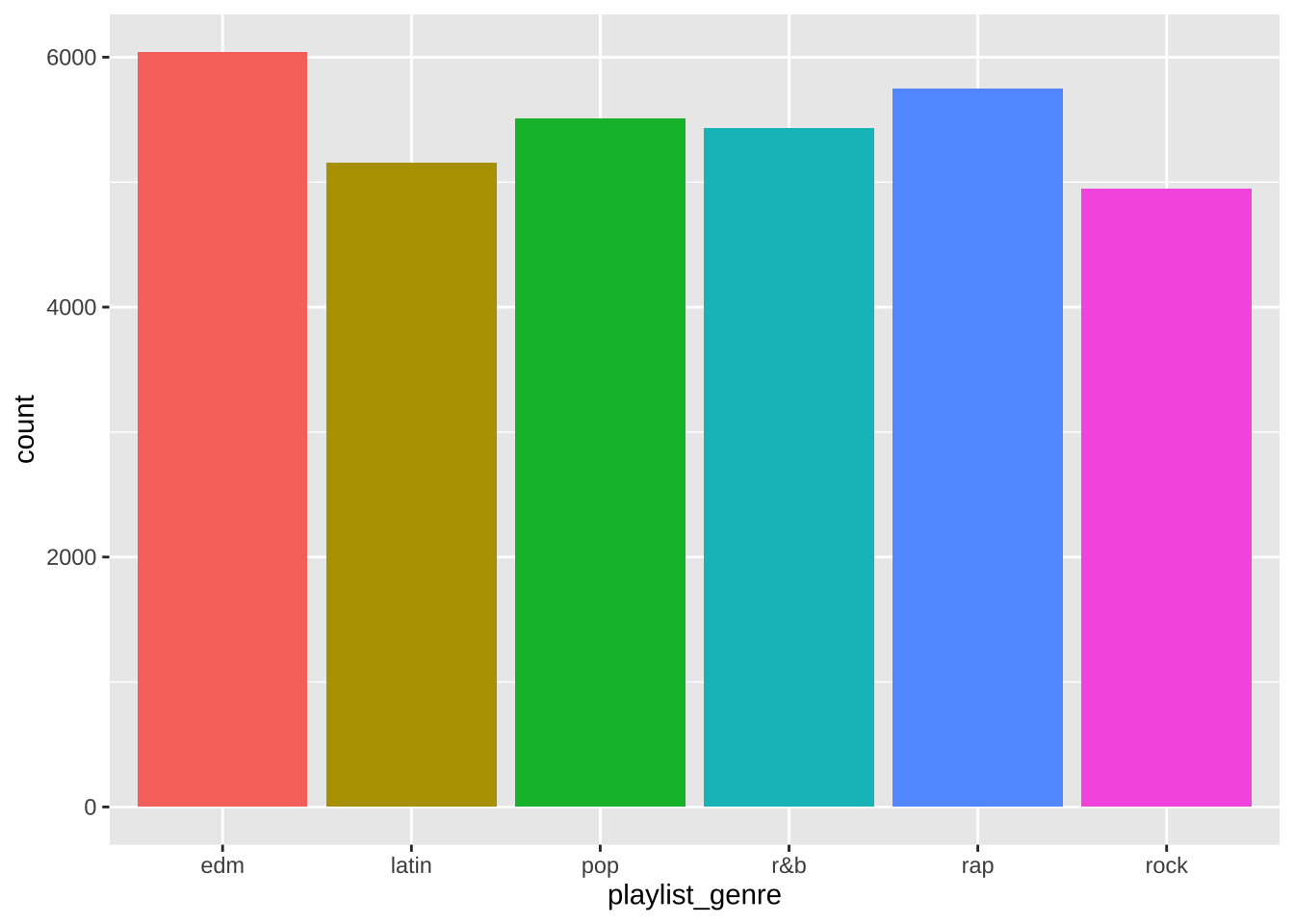## Change legend title and labels

`scale_fill_discrete(name = "New Legend Title", labels = c("lab1" = "Label 1", "lab2" = "Label 2"))`

Alright, say I do want the legend. How do I make it something readable?

``````spotify_songs %>%
ggplot(aes(x = playlist_genre, fill = playlist_genre)) +
geom_histogram(stat = "count") +
scale_fill_discrete(name = "Playlist Genre",
labels = c("edm" = "EDM",
"latin" = "Latin",
"pop" = "Pop",
"r&b" = "R&B",
"rap" = "Rap",
"rock" = "Rock"))``````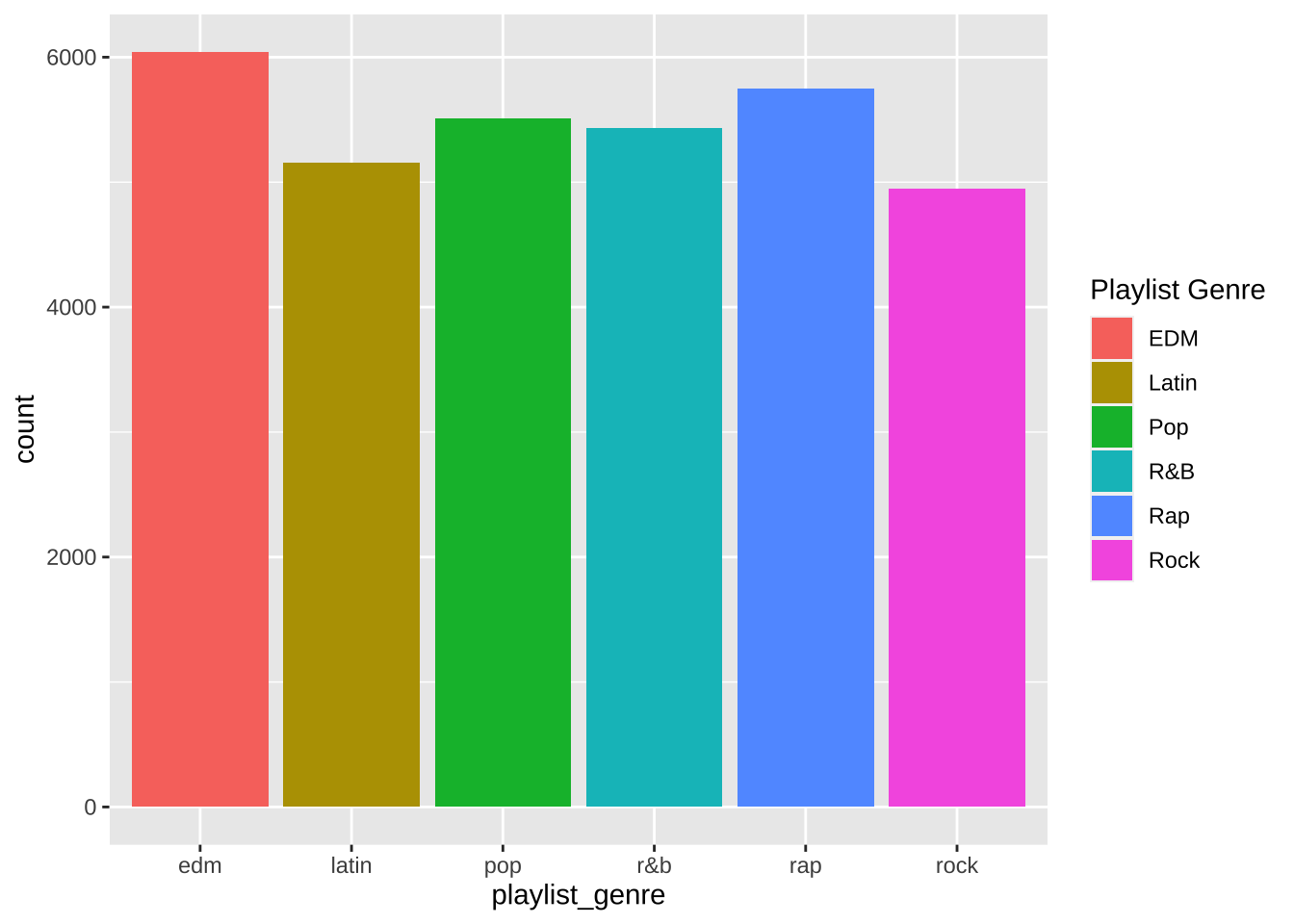## Manually change colors

`scale_fill_manual("New Legend Title", values = c("lab1" = "#000000", "lab2" = "#FFFFFF"))`

This is a bit trickier, in that you cannot use `scale_fill_manual` and `scale_fill_discrete` separately on the same plot as they override each other. However, if you want to change the labels and the colors together, you can use `scale_fill_manual` like below.

``````spotify_songs %>%
ggplot(aes(x = playlist_genre, fill = playlist_genre)) +
geom_histogram(stat = "count") +
scale_fill_manual(name = "Playlist Genre",
labels = c("edm" = "EDM",
"latin" = "Latin",
"pop" = "Pop",
"r&b" = "R&B",
"rap" = "Rap",
"rock" = "Rock"),
values = c("edm" = "#0081e8",
"latin" = "#9597f0",
"pop" = "#d4b4f6",
"r&b" = "#ffd6ff",
"rap" = "#ffa1d4",
"rock" = "#ff688c"))``````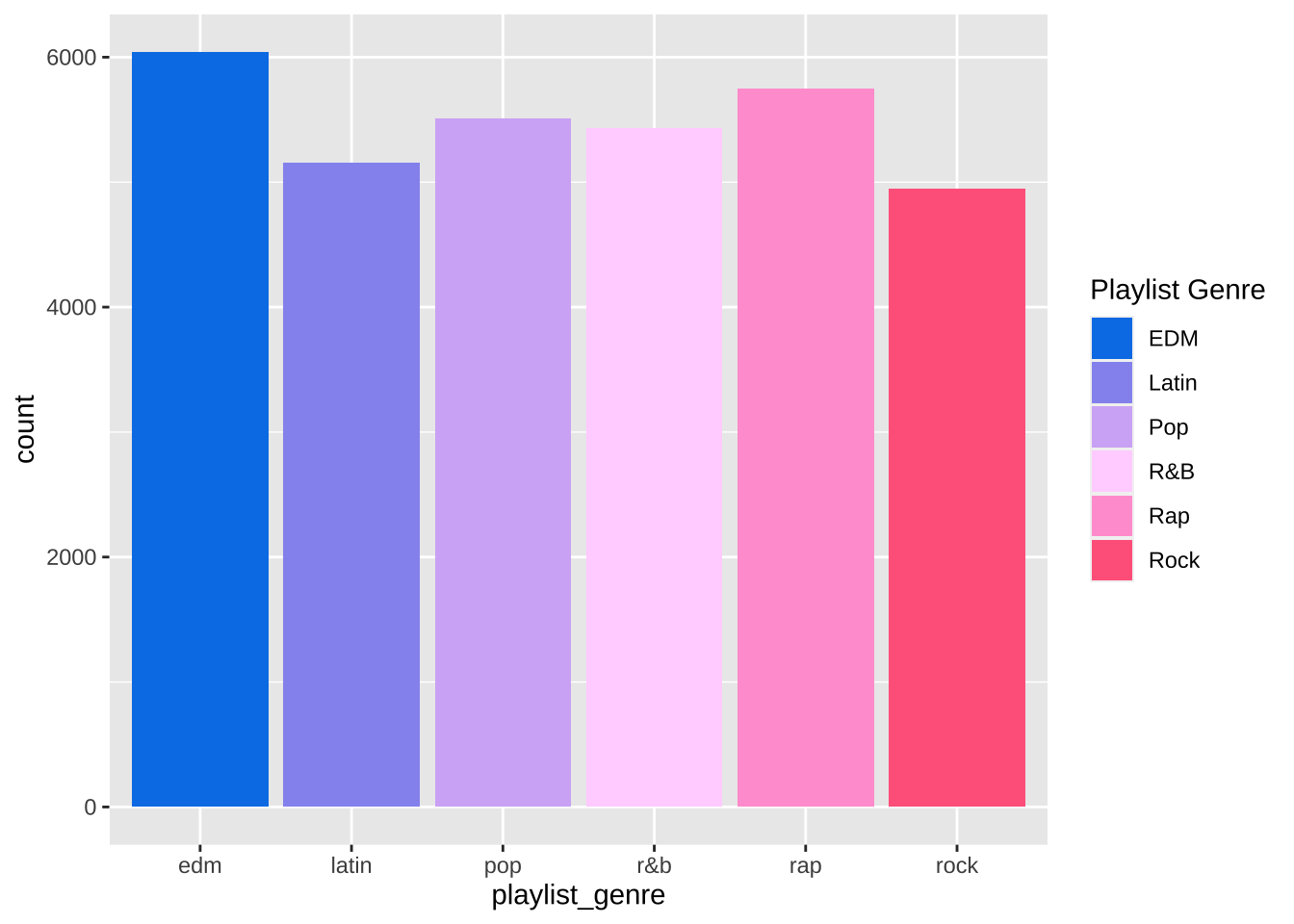## Remove x-axis labels

`theme(axis.title.x = element_blank(), axis.text.x = element_blank(), axis.ticks.x = element_blank())`

In this case, since we have a legend, we don’t need any x axis labels. Sometimes I use this if there’s redundant information or if it otherwise makes the graph look cleaner.

``````spotify_songs %>%
ggplot(aes(x = playlist_genre, fill = playlist_genre)) +
geom_histogram(stat = "count") +
scale_fill_manual(name = "Playlist Genre",
labels = c("edm" = "EDM",
"latin" = "Latin",
"pop" = "Pop",
"r&b" = "R&B",
"rap" = "Rap",
"rock" = "Rock"),
values = c("edm" = "#0081e8",
"latin" = "#9597f0",
"pop" = "#d4b4f6",
"r&b" = "#ffd6ff",
"rap" = "#ffa1d4",
"rock" = "#ff688c")) +
theme(axis.title.x = element_blank(),
axis.text.x = element_blank(),
axis.ticks.x = element_blank())``````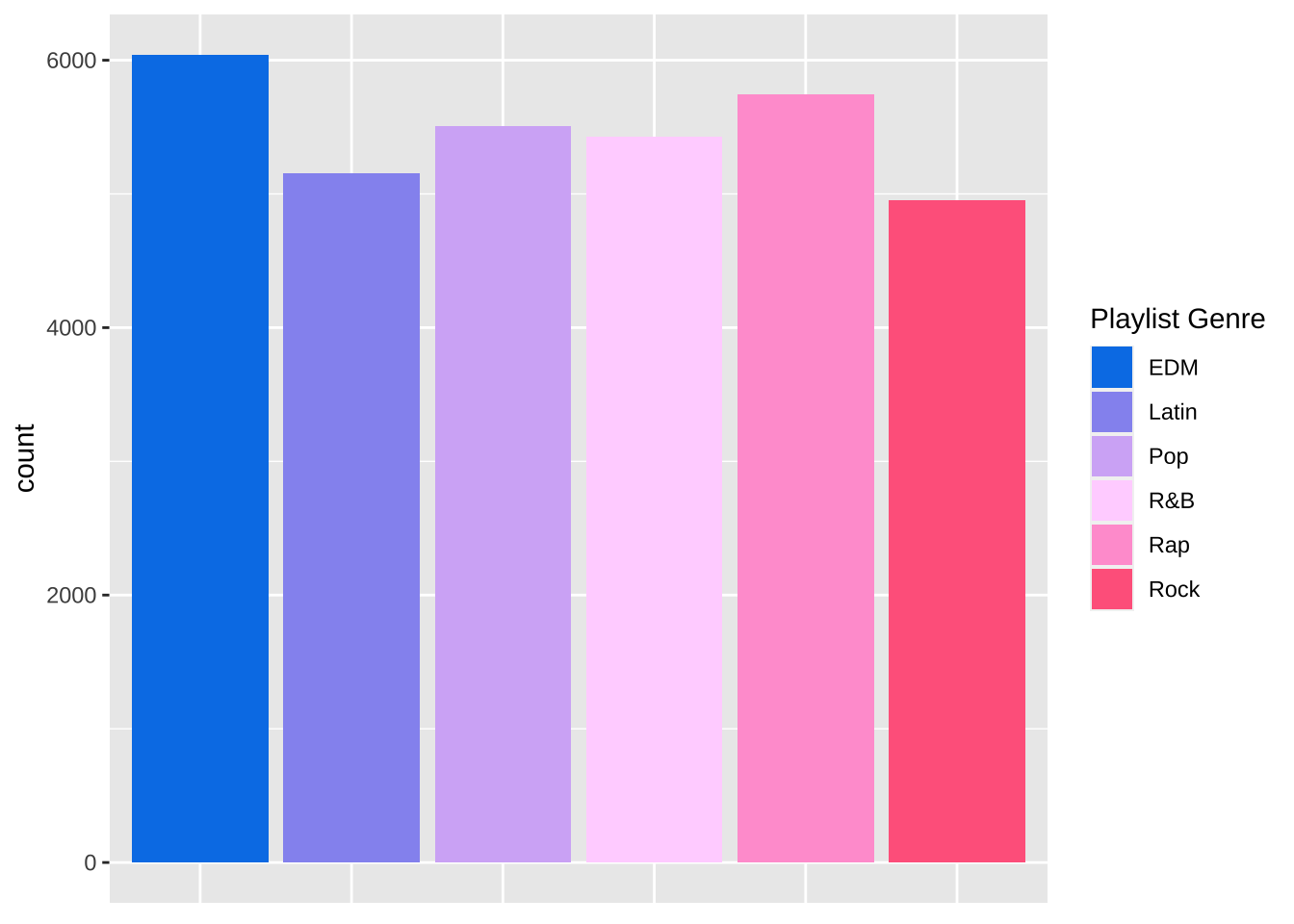## Start the y-axis at a specific number

`scale_y_continuous(name = "New Y Axis Title", limits = c(0, 1000000))`

Often times, we want our graph’s y axis to start at 0. In this example it already does, but this handy parameter allows us to set exactly what we want our y axis to be.

``````spotify_songs %>%
ggplot(aes(x = playlist_genre, fill = playlist_genre)) +
geom_histogram(stat = "count") +
scale_fill_manual(name = "Playlist Genre",
labels = c("edm" = "EDM",
"latin" = "Latin",
"pop" = "Pop",
"r&b" = "R&B",
"rap" = "Rap",
"rock" = "Rock"),
values = c("edm" = "#0081e8",
"latin" = "#9597f0",
"pop" = "#d4b4f6",
"r&b" = "#ffd6ff",
"rap" = "#ffa1d4",
"rock" = "#ff688c")) +
theme(axis.title.x = element_blank(),
axis.text.x = element_blank(),
axis.ticks.x = element_blank()) +
scale_y_continuous(name = "Count", limits = c(0, 10000))``````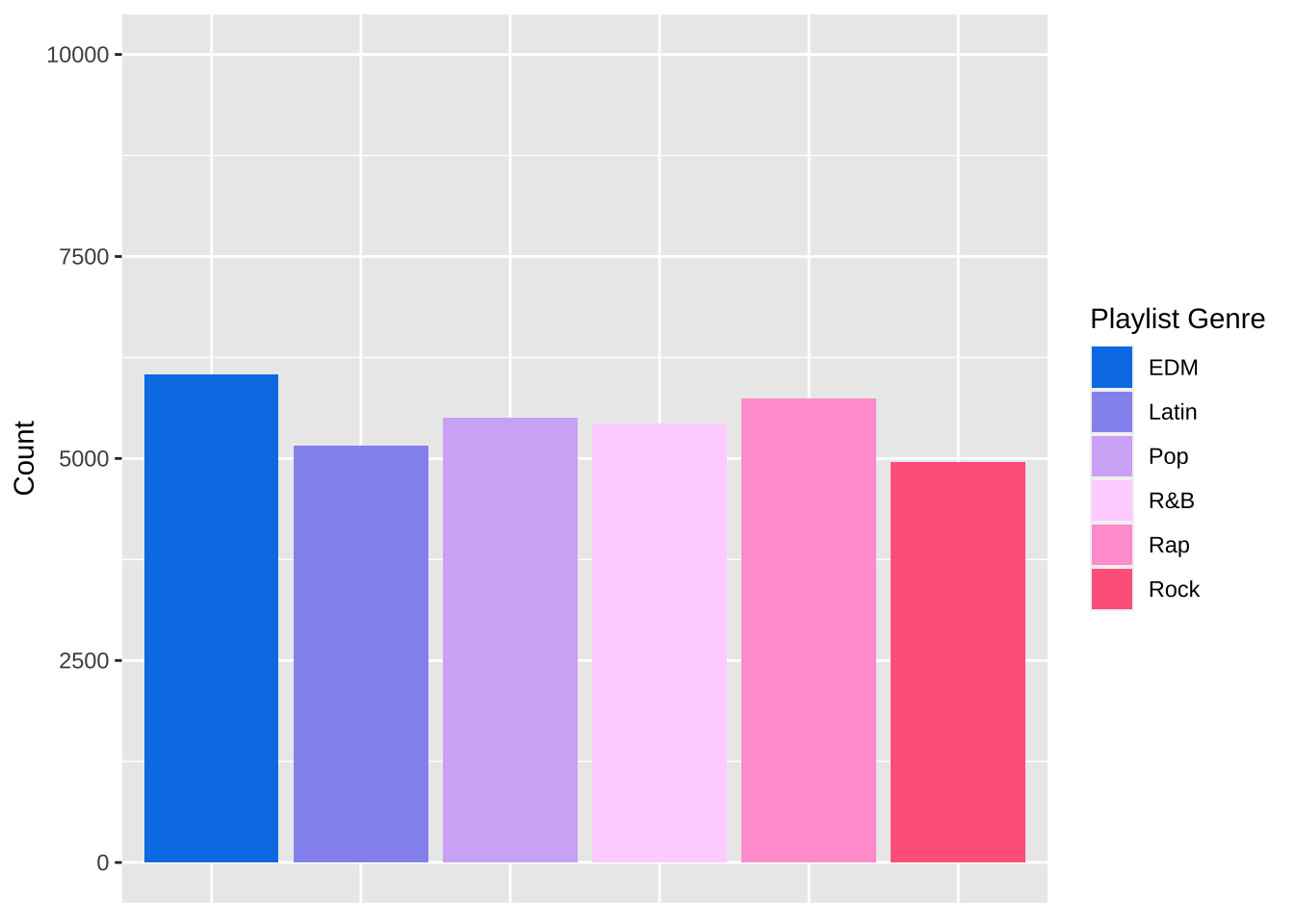## Use scales on the y-axis

`scale_y_continuous(label = scales::format)`

Depending on our data, we may want the y axis to be formatted a certain way (using dollar signs, commas, percentage signs, etc.). The handy {scales} package allows us to do that easily.

``````spotify_songs %>%
ggplot(aes(x = playlist_genre, fill = playlist_genre)) +
geom_histogram(stat = "count") +
scale_fill_manual(name = "Playlist Genre",
labels = c("edm" = "EDM",
"latin" = "Latin",
"pop" = "Pop",
"r&b" = "R&B",
"rap" = "Rap",
"rock" = "Rock"),
values = c("edm" = "#0081e8",
"latin" = "#9597f0",
"pop" = "#d4b4f6",
"r&b" = "#ffd6ff",
"rap" = "#ffa1d4",
"rock" = "#ff688c")) +
theme(axis.title.x = element_blank(),
axis.text.x = element_blank(),
axis.ticks.x = element_blank()) +
scale_y_continuous(name = "Count", limits = c(0, 10000),
labels = scales::comma)``````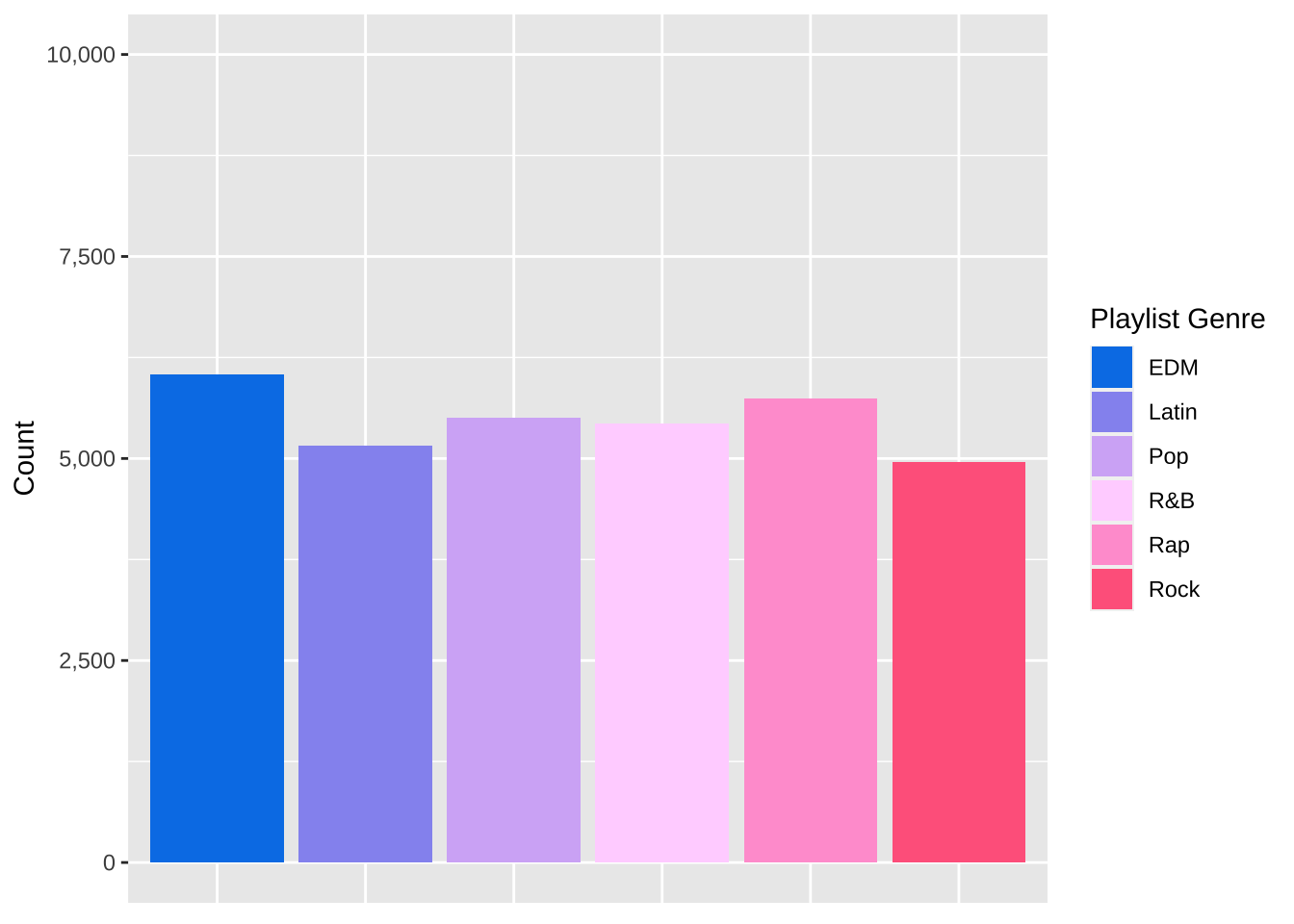There we have it! Six things I always eventually end up Googling when I am making plots using {ggplot2}. Hopefully now I can just look at this page instead of searching each and every time!

Liked this post? I’d love for you to retweet!